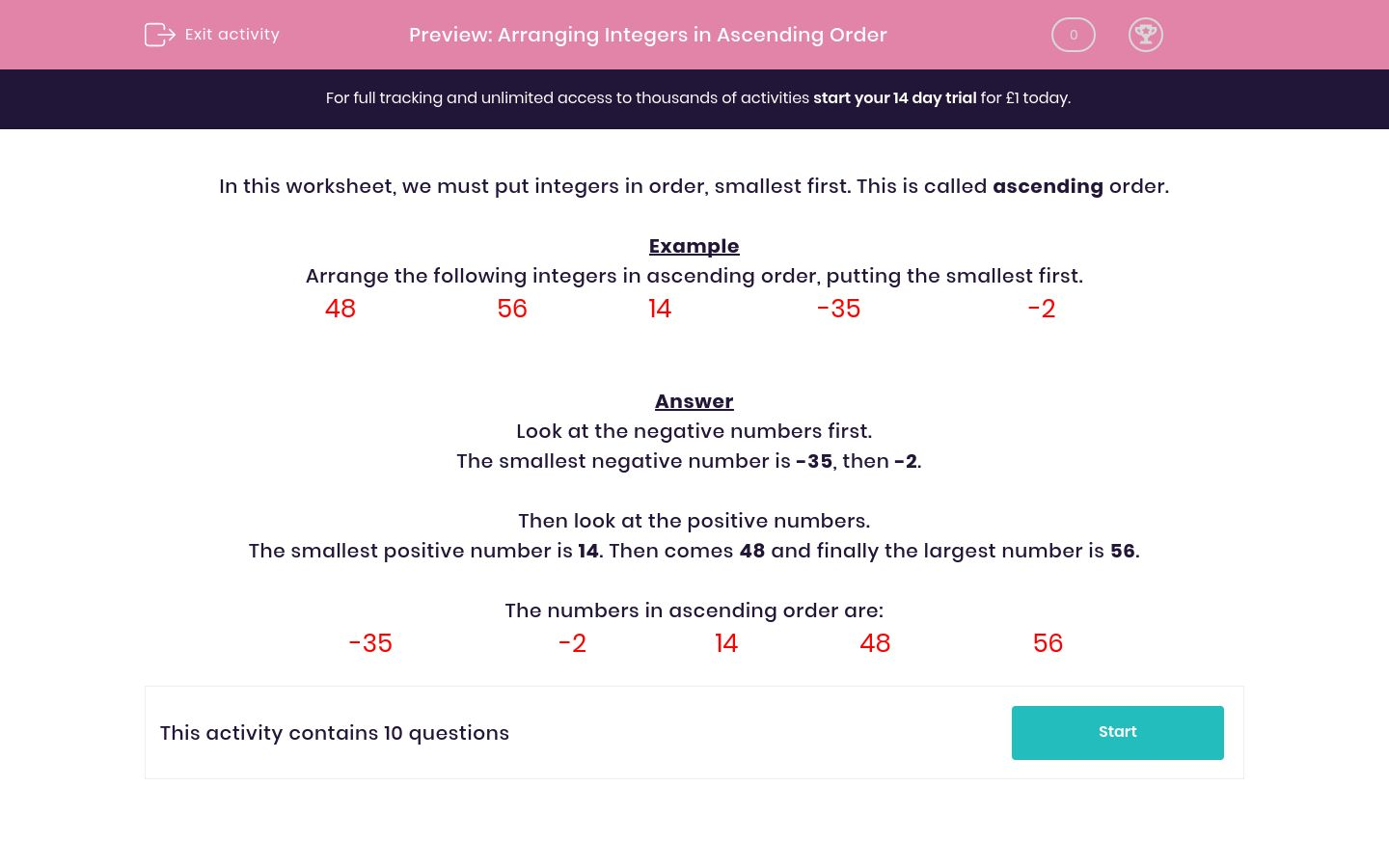# Arranging Integers in Ascending Order

In this worksheet, students have to arrange positive and negative integers in ascending order of size.Key stage:  KS 2

Curriculum topic:   Maths and Numerical Reasoning

Curriculum subtopic:   Place Value

Difficulty level:#### Worksheet Overview

In this worksheet, we must put integers in order, smallest first. This is called ascending order.

Example

Arrange the following integers in ascending order, putting the smallest first.

 48 56 14 -35 -2

Look at the negative numbers first.

The smallest negative number is -35, then -2.

Then look at the positive numbers.

The smallest positive number is 14. Then comes 48 and finally the largest number is 56.

The numbers in ascending order are:

 -35 -2 14 48 56

### What is EdPlace?

We're your National Curriculum aligned online education content provider helping each child succeed in English, maths and science from year 1 to GCSE. With an EdPlace account you’ll be able to track and measure progress, helping each child achieve their best. We build confidence and attainment by personalising each child’s learning at a level that suits them.

Get started